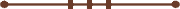####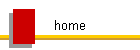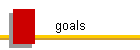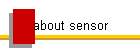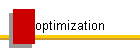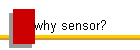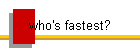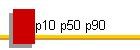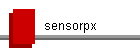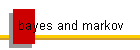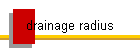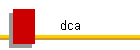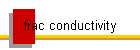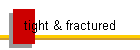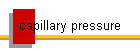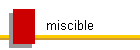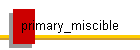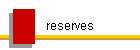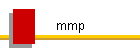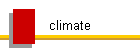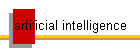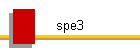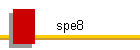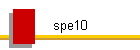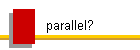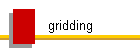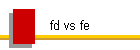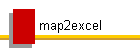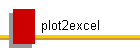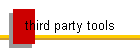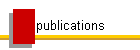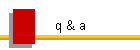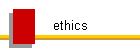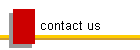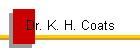#### SensorPx

SensorPx computes probabilistic Px,y,z production/injection results from any given set of fort.61 binary plot file production/injection results obtained from a set of Sensor runs having equally probable descriptions.  The case results are first interpolated to a set of common times and the cases are then ranked in each variable at each common time to determine and output the Px, Py, and Pz values in standard Sensor fort.61 plot file format, for easy viewing/plotting/computing in any Sensor-compatible post-processor or workflow.  The results are  written as 3 separate files - casename.px, casename.py, and casename.pz, where casename is the name of the SensorPx input data file casename.spx, and x,y, and z are the specified percentiles for use in calculating the probabilistic results.  Additional output includes printed tables of Px, Py, and Pz Field production/injection vs. time results for easy import into economics packages and spreadsheets.

Either exceedance probabilities (variable value is greater than or equal to the Px value in at least x% of cases) or cumulative probabilities (variable value is less than or equal to the Px value in at least x% of cases) can be computed.

At least 10 Sensor runs are required, but far more than that are usually required for statistically significant results, depending on the number of unknown variables and their variation, and their effect on results.  A set of results from some number of cases is statistically significant if the probabilistic results do not change significantly as additional cases are added, or if multiple sets of the same number of random realizations give essentially the same probabilistic results.  If a set of results is statistically significant, then probabilistic forecasts are reliable if all assumptions regarding the identity and variability of unknowns are correct, if Sensor model assumptions are correct, and if numerical dispersion is properly controlled in the Sensor runs.  While a statistically significant set of results is generally required for reliable uncertainty quantification in production forecasts, a set of n results (that may not be statistically significant) is sufficient for use in optimization if the operational alternatives have a significantly greater effect on those results than the errors in the probabilistic forecasts, which can be estimated by observed variation of the results of multiple sets of n runs, and which decrease with increasing n.  This is demonstrated by the examples below.

SensorPx is available for integration with custom workflows and Sensor-compatible pre-processors.  A companion program, Makespx, provides a simple workflow to  create batch files that execute a set of specified equally-probable Sensor runs (datafiles), rename and collect the fort.61 results files, create the SensorPx data file, and launch SensorPx to compute the probabilistic results.  The Sensor runs are executed in any specified number of simultaneous sets of serial runs in order to maximize system output and efficiency.

A node-locked Sensor license makes probabilistic forecasting economically possible because it does not limit the number of simultaneous users or runs, allowing you to make use of 100% of the capabilities of your computer.  The number of simultaneous runs is limited only by machine memory.

For simple (Cartesian) systems, equally-probable sets of Sensor runs can be generated by Sensor using the Uncertain Inputs features documented here.  In more complex cases, the sets of Sensor data or results files must be generated by a preprocessor or custom workflow, for input to Makespx and/or SensorPx.

Makeoptdat is another program that can be used to make more rigorous optimizations, requiring fewer numbers of runs, by generating the same sets of realizations of the unknowns for any number of operational options.  The data uncertainties must be represented by the Sensor Uncertain Inputs features, which limits the application to simple systems with Cartesian grids.  Similar functions may be provided by custom workflows or preprocessors integrated with Makespx or SensorPx.  Makeoptdat automatically creates the equivalent sets of realizations for each operational option and creates the Makespx input data files for each option.  Execution of  Makespx (and the created batch files) for each option then provides for rigorous computation and comparison of probabilistic results.  The probability that option 1 is better than option 2 can be estimated as the fraction of total realizations in which option 1 outperforms option 2.  The accuracy of the estimate is indicated by its change with the number of realizations considered.  This is demonstrated in Chapter 5, Examples 2 and 3.

Uses of SensorPx, Makespx, and Makeoptdat include:

• Generation of probabilistic forecasts from any given set of equally-probable Sensor run outputs created by any workflow.

• Probabilistic appraisal, forecasting, and optimization for cases and workflows where no production history is available, or where many history-matched models are generated or available.

• Generation, execution, and evaluation of unlimited numbers of equally probable realizations from each Sensor data file that uses Uncertain Inputs - specified probabilistic input distributions for the most common unknowns that are populated using random number generation.  Each execution of Sensor on the same data file using Uncertain Inputs gives a different (but reproducible) equally-probable combination (realization) of the uncertain variables.  Probabilistic results are determined for any specified number of automatically-generated, equally-probable realizations representing any specified set of estimated input probabilistic distributions of the independent uncertainties in reservoir description.  These realizations can also be very effectively used as starting point inputs to assisted history-matching workflows in finding the large number of matches needed to quantify uncertainty in predictions and optimizations that are made using them.

## PERFORMANCE

In our spe1-based examples of our simple probabilistic workflows, we're running 10,000 realizations (in 8 parallel sets of 1250 serial runs) in less than 10 minutes using our commercial simulator on a typical desktop (machine 3 on our BENCHMARKS page).

© 2000 - 2022 Coats Engineering, Inc.